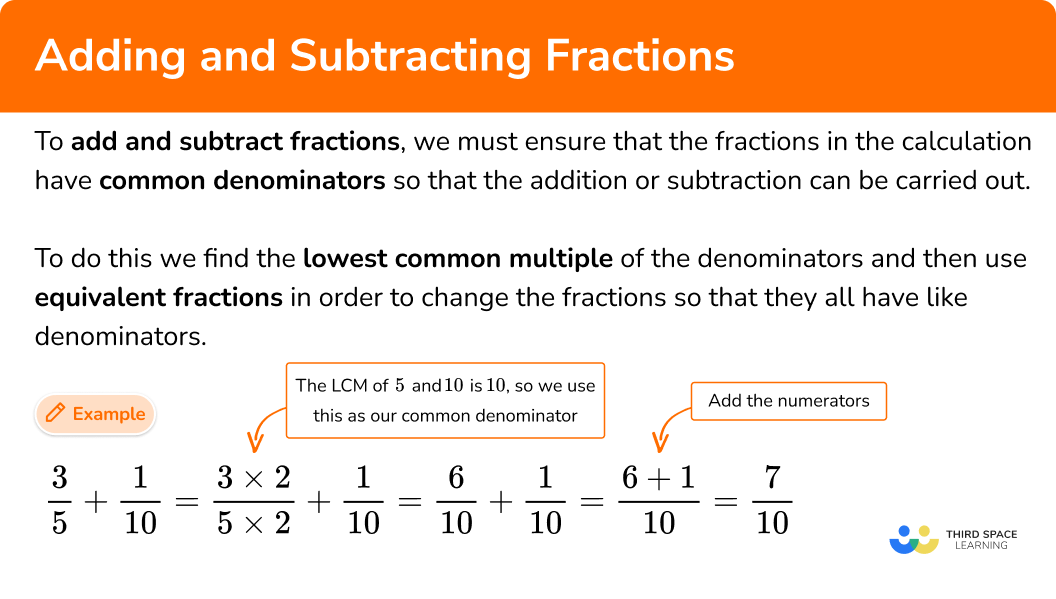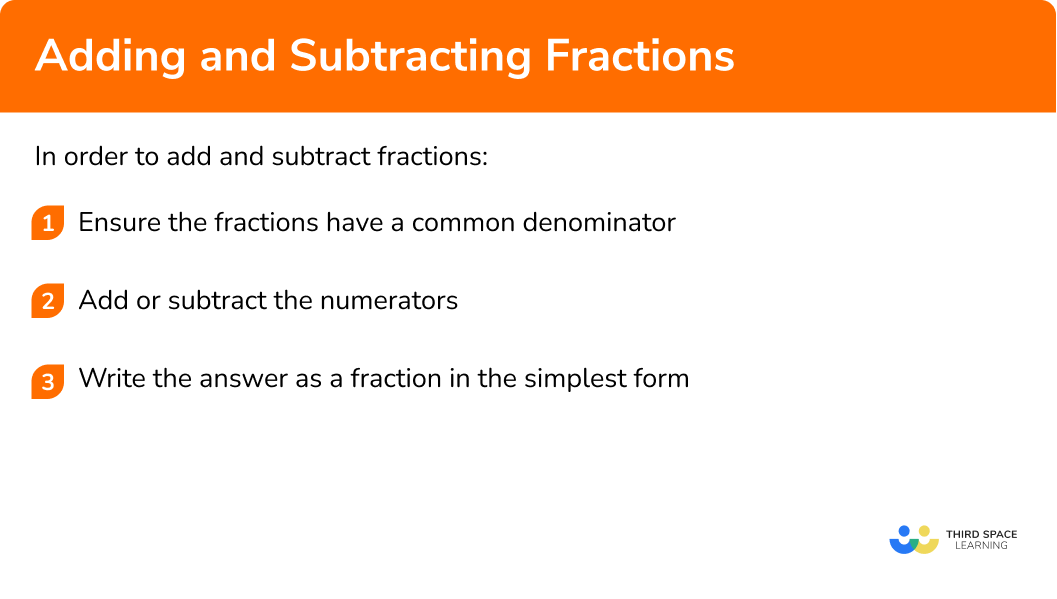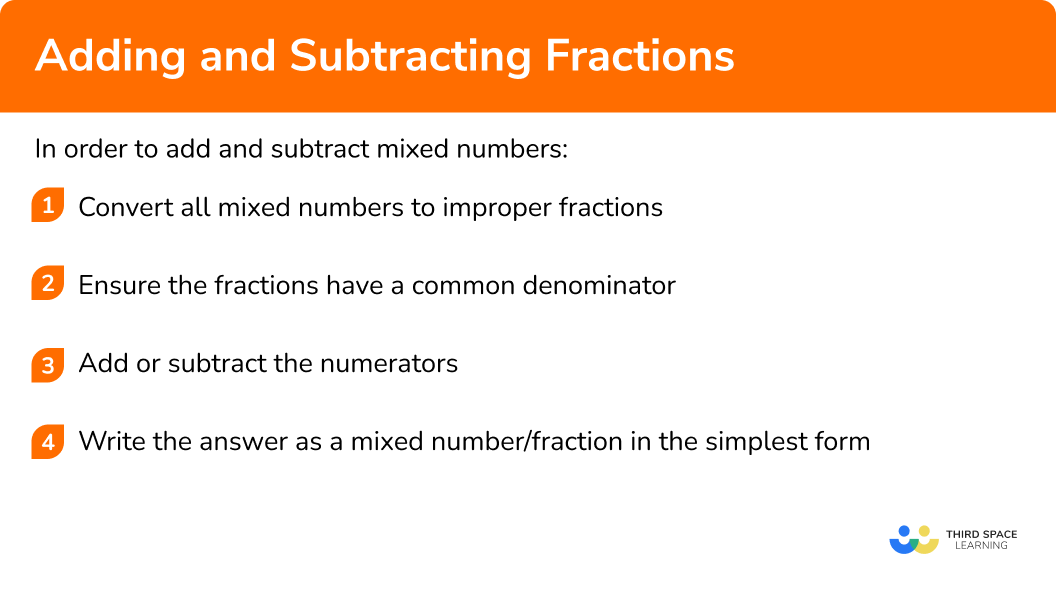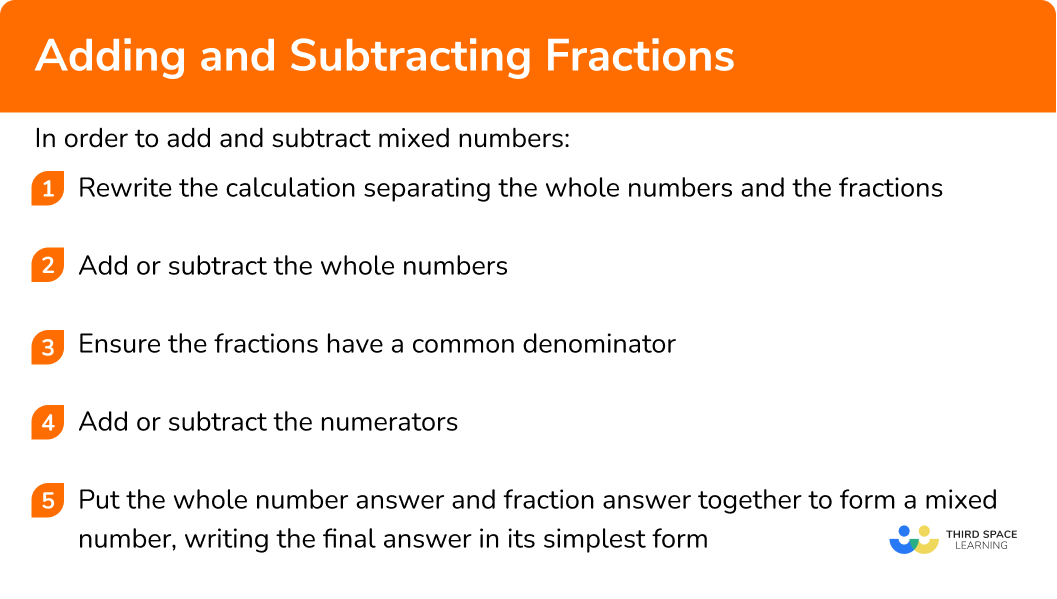GCSE Maths Number FDP Fractions

Here we will learn about adding and subtracting fractions, including when the fractions have the same denominator, when they have different denominators and when they are mixed numbers.

There are also adding and subtracting fractions worksheets based on Edexcel, AQA and OCR exam questions, along with further guidance on where to go next if you’re still stuck.

## What is adding and subtracting fractions?

Adding and subtracting fractions is the skill of ensuring the fractions in a calculation have common denominators so that the addition or subtraction can be carried out.

A fraction is a numerical quantity that is not a whole number (integer) but can be expressed as one integer divided by another. The division is represented by a horizontal line.

The integer on the top of the line is called the numerator.

The integer underneath the line is called the denominator.

If the fractions within the question have the same denominators already, then the numerators can be easily added/subtracted and the final answer will also have the same denominator.

For example,

\cfrac{5}{7} - \cfrac{2}{7} = \cfrac{5-2}{7} = \cfrac{3}{7}

If the fractions within the question have different denominators, we must use equivalent fractions along with knowledge of calculating the lowest common multiple to change the fractions so that they have like denominators.

For example,

\cfrac{3}{5} + \cfrac{1}{10} = \cfrac{3\times 2}{5\times 2} + \cfrac{1}{10} =\cfrac{6}{10} + \cfrac{1}{10} = \cfrac{6+1}{10} = \cfrac{7}{10}

Step-by-step guide: Subtracting fractions

### What is adding and subtracting fractions?## How to add and subtract fractions

In order to add and subtract fractions:

1. Ensure the fractions have a common denominator.
2. Add or subtract the numerators.
3. Write the answer as a fraction in the simplest form.

### Explain how to add and subtract fractions### Related lessons on fractions

Adding and subtracting fractions is part of our series of lessons to support revision on fractions. You may find it helpful to start with the main fractions lesson for a summary of what to expect, or use the step by step guides below for further detail on individual topics. Other lessons in this series include:

## Adding and subtracting fractions examples

### Example 1: adding fractions with a common denominator

Determine the value of \; \cfrac{5}{9}+\cfrac{2}{9}.

1. Ensure the fractions have a common denominator.

The fractions have a common denominator of 9.

\cfrac{5}{9}+\cfrac{2}{9}=\cfrac{5+2}{9}=\cfrac{7}{9}.

3Write the answer as a fraction in the simplest form.

7 and 9 do not share any common factors except 1 and so the fraction is already in its simplest form.

The solution is \cfrac{7}{9}.

### Example 2: adding fractions where one denominator is a multiple of the other

Determine the value of \; \cfrac{3}{8}+\cfrac{1}{4}.

Ensure the fractions have a common denominator.

Write the answer as a fraction in the simplest form.

### Example 3: adding fractions with different denominators

Determine the value of \; \cfrac{1}{2}+\cfrac{5}{11}.

Ensure the fractions have a common denominator.

Write the answer as a fraction in the simplest form.

### Example 4: subtracting fractions with a common denominator

Determine the value of \; \cfrac{7}{12}-\cfrac{5}{12}.

Ensure the fractions have a common denominator.

Write the answer as a fraction in the simplest form.

### Example 5: subtracting fractions where one denominator is a multiple of the other

Determine the value of \; \cfrac{11}{15}-\cfrac{2}{5}.

Ensure the fractions have a common denominator.

Write the answer as a fraction in the simplest form.

### Example 6: subtracting fractions with different denominators

Determine the value of \; \cfrac{5}{6}-\cfrac{7}{9}.

Ensure the fractions have a common denominator.

Write the answer as a fraction in the simplest form.

## How to add and subtract mixed numbers

In order to add and subtract mixed numbers:

1. Convert all mixed numbers to improper fractions.
2. Ensure the fractions have a common denominator.
3. Add or subtract the numerators.
4. Write the answer as a mixed number/fraction in the simplest form.

### Explain how to add and subtract mixed numbers### Example 7: adding mixed numbers with different denominators

Determine the value of \; 1\cfrac{3}{4}+2\cfrac{5}{6}.

Convert all mixed numbers to improper fractions.

Ensure the fractions have a common denominator.

Write the answer as a fraction in the simplest form.

### Example 8: subtracting mixed numbers with different denominators

Determine the value of \; 3\cfrac{1}{2}-1\cfrac{2}{3}.

Convert all mixed numbers to improper fractions.

Ensure the fractions have a common denominator.

Write the answer as a fraction in the simplest form.

### Example 9: adding and subtracting mixed numbers with different denominators

Determine the value of \; 4\cfrac{2}{3}+2\cfrac{4}{5}-1\cfrac{5}{6}.

Convert all mixed numbers to improper fractions.

Ensure the fractions have a common denominator.

Write the answer as a fraction in the simplest form.

## How to add and subtract mixed numbers (alternative method)

In order to add and subtract mixed numbers:

1. Rewrite the calculation separating the whole numbers and the fractions.
2. Add or subtract the whole numbers.
3. Ensure the fractions have a common denominator.
4. Add or subtract the numerators.
5. Put the whole number answer and fraction answer together to form a mixed number writing the final answer in the simplest form.

### Explain how to add and subtract mixed numbers (alternative method)### Example 10: adding and subtracting mixed numbers with common denominators

Determine the value of \; 5\cfrac{2}{7}+3\cfrac{4}{7}-4\cfrac{5}{7}.

Rewrite the calculation separating the whole numbers and the fractions.

Add or subtract the whole numbers.

Ensure the fractions have a common denominator.

Put the whole number answer and fraction answer together to form a mixed number writing the final answer in the simplest form.

### Example 11: adding mixed numbers where one denominator is a multiple of the other

Determine the value of \; 1\cfrac{2}{3}+6\cfrac{10}{21}.

Rewrite the calculation separating the whole numbers and the fractions.

Add or subtract the whole numbers.

Ensure the fractions have a common denominator.

Put the whole number answer and fraction answer together to form a mixed number writing the final answer in the simplest form.

### Example 12: subtracting mixed numbers with different denominators

Determine the value of \; 5\cfrac{1}{10}-2\cfrac{7}{15}.

Rewrite the calculation separating the whole numbers and the fractions.

Add or subtract the whole numbers.

Ensure the fractions have a common denominator.

Put the whole number answer and fraction answer together to form a mixed number writing the final answer in the simplest form.

### Common misconceptions

Sometimes students will make the following kind of mistake.

\cfrac{2}{9} + \cfrac{5}{9} = \cfrac{2+5}{9+9} = \cfrac{7}{18}

They will check that denominators are the same before adding the fractions but then they will still add the denominators together.

This is incorrect. When adding fractions with a common denominator we only add the numerators, keeping the denominator the same, like this.

\cfrac{2}{9} + \cfrac{5}{9} = \cfrac{2+5}{9} = \cfrac{7}{9}

Similarly, students might make the following kind of mistake when subtracting fractions.

\cfrac{5}{7}-\cfrac{2}{7} = \cfrac{5-2}{7-7} = \cfrac{3}{0}

This is incorrect. When subtracting fractions with a common denominator we only subtract the numerators, keeping the denominator the same, like this.

\cfrac{5}{7}-\cfrac{2}{7} = \cfrac{5-2}{7} = \cfrac{3}{7}

• Forgetting to make the denominators the same

Sometimes students will make the following kind of mistake.

\cfrac{2}{3} + \cfrac{3}{5} = \cfrac{2+3}{3+5} = \cfrac{5}{8}

This is incorrect. When fractions are added/subtracted they must have the same denominator. If the denominators are not the same, they must be converted first, using equivalent fractions. The correct method is,

\cfrac{2}{3} + \cfrac{3}{5} = \cfrac{2\times 5}{3\times 5} + \cfrac{3\times 3}{5\times 3} = \cfrac{10}{15} + \cfrac{9}{15} = \cfrac{10+9}{15} = \cfrac{19}{15} = 1\cfrac{4}{15}

• Forgetting to change the numerator as well as the denominator when writing an equivalent fraction in a calculation

Sometimes students will make the following kind of mistake.

\cfrac{2}{7} + \cfrac{4}{21} = \cfrac{2}{7\times 3} + \cfrac{4}{21} = \cfrac{2}{21} + \cfrac{4}{21}

Here the student has attempted to make the denominators the same by multiplying the denominator of 7 by 3. However, they have forgotten to also multiply the numerator by 3, so the equivalent fraction is incorrect.

The correct equivalent fraction would be \cfrac{6}{21}.

Similarly some students might make the following kind of mistake.

\cfrac{4}{5}-\cfrac{2}{7} = \cfrac{4}{5\times 7}-\cfrac{2}{7\times 5} = \cfrac{4}{35}-\cfrac{2}{35}

Here the student has correctly identified that the lowest common multiple of the denominators 5 and 7 is 35.

However, they have focussed on changing the denominators only and have forgotten to change the numerators as well to ensure the new fractions are equivalent. The correct method is,

\cfrac{4}{5}-\cfrac{2}{7} = \cfrac{4\times 7}{5\times 7}-\cfrac{2\times 5}{7\times 5} = \cfrac{28}{35}-\cfrac{10}{35}

### Practice adding and subtracting fractions questions

1. Determine the value of \; \cfrac{3}{7}+\cfrac{2}{7}.

\cfrac{5}{14}\cfrac{35}{49}\cfrac{5}{7}\cfrac{10}{9}\cfrac{3}{7}+\cfrac{2}{7}=\cfrac{3+2}{7}=\cfrac{5}{7}

2. Determine the value of \; \cfrac{5}{9}+\cfrac{3}{18}.

\cfrac{13}{18}\cfrac{8}{27}\cfrac{8}{18}\cfrac{8}{36}\cfrac{5}{9}+\cfrac{3}{18}=\cfrac{10}{18}+\cfrac{3}{18}=\cfrac{10+3}{18}=\cfrac{13}{18}

3. Determine the value of \; \cfrac{6}{7}+\cfrac{4}{9}.

\cfrac{10}{16}5\cfrac{1}{8}\cfrac{10}{63}1\cfrac{19}{63}\cfrac{6}{7}+\cfrac{4}{9}=\cfrac{54}{63}+\cfrac{28}{63}=\cfrac{54+28}{63}=\cfrac{82}{63}=1\cfrac{19}{63}

4. Determine the value of \; \cfrac{9}{10}-\cfrac{3}{10}.

6\cfrac{3}{5}\cfrac{6}{0}\cfrac{6}{20}\cfrac{9}{10}-\cfrac{3}{10}=\cfrac{9-3}{10}=\cfrac{6}{10}=\cfrac{3}{5}

5. Determine the value of \; \cfrac{5}{12}-\cfrac{1}{4}.

\cfrac{1}{2}\cfrac{1}{6}\cfrac{1}{3}\cfrac{1}{11}\cfrac{5}{12}-\cfrac{1}{4}=\cfrac{5}{12}-\cfrac{3}{12}=\cfrac{5-3}{12}=\cfrac{2}{12}=\cfrac{1}{6}

6. Determine the value of \; \cfrac{12}{13}-\cfrac{2}{3}.

1\cfrac{10}{13}\cfrac{-15}{26}\cfrac{10}{39}\cfrac{12}{13}-\cfrac{2}{3}=\cfrac{36}{39}-\cfrac{26}{39}=\cfrac{36-26}{39}=\cfrac{10}{39}

7. Determine the value of \; 2\cfrac{1}{5}+3\cfrac{2}{5}.

5\cfrac{3}{10}\cfrac{4}{5}5\cfrac{3}{5}8\cfrac{1}{5}\begin{aligned} &2\cfrac{1}{5}+3\cfrac{2}{5}\\\\ &=2+3+\cfrac{1}{5}+\cfrac{2}{5}\\\\ &=5+\cfrac{1+2}{5}\\\\ &=5+\cfrac{3}{5}\\\\ &=5\cfrac{3}{5} \end{aligned}

8. Determine the value of \; 4\cfrac{1}{3}-1\cfrac{5}{6}.

3\cfrac{4}{3}2\cfrac{1}{3}2\cfrac{1}{2}3\cfrac{1}{2}\begin{aligned} &4\cfrac{1}{3}-1\cfrac{5}{6}\\\\ &=\cfrac{13}{3}-\cfrac{11}{6}\\\\ &=\cfrac{26}{6}-\cfrac{11}{6}\\\\ &=\cfrac{26-11}{6}\\\\ &=\cfrac{15}{6}\\\\ &=\cfrac{5}{2}\\\\ &=2\cfrac{1}{2} \end{aligned}

### Adding and subtracting fractions GCSE questions

1. Adam eats \cfrac{1}{5} of a pizza. Ben eats \cfrac{1}{4}. What fraction of the pizza is left?

(2 marks)

\cfrac{1}{5}+\cfrac{1}{4}=\cfrac{4}{20}+\cfrac{5}{20}\left( =\cfrac{9}{20}\right)

(1)

1-\cfrac{9}{20}\left( =\cfrac{11}{20}\right)

(1)

2. Frazer is travelling to Paris. It takes him 2\cfrac{2}{3} hours to drive from Bury St Edmunds to Dover.

The crossing from Dover to Calais takes 1\cfrac{1}{2} hours.

The drive from Calais to Paris takes 3\cfrac{3}{4} hours.

What was the total time of Frazer’s journey? Give your answer in hours.

(2 marks)

2\cfrac{2}{3}+1\cfrac{1}{2}+3\cfrac{3}{4}
2\cfrac{8}{12}+1\cfrac{6}{12}+3\cfrac{9}{12}

(1)

6+\cfrac{8+6+9}{12}=6+\cfrac{23}{12}=6+1\cfrac{11}{12}
=7\cfrac{11}{12} hours

(1)

3. Urma has \cfrac{4}{5} of a litre tin of red paint. She also has \cfrac{5}{8} of a litre tin of yellow paint.

She mixes the paint together to make orange paint. Urma paints a wall which uses \cfrac{3}{4} of a litre of orange paint.

Urma wants to paint another wall of the same size. Does she have enough orange paint left to do this?

(4 marks)

\cfrac{4}{5}+\cfrac{5}{8}-\cfrac{3}{4}

(1)

\cfrac{32}{40}+\cfrac{25}{40}-\cfrac{30}{40}

(1)

\cfrac{32+25-30}{40}=\cfrac{27}{40}

(1)

\cfrac{3}{4}=\cfrac{30}{40} \cfrac{30}{40}>\cfrac{27}{40}

No, Urma does not have enough orange paint to paint the second wall.

(1)

## Learning checklist

You have now learned how to:

• Add and subtract fractions with the same denominator
• Add and subtract fractions with different denominators and mixed numbers, using the concept of equivalent fractions
• Calculate exactly with fractions
• Use formal written methods to show your working out when calculating with fractions

## Still stuck?

Prepare your KS4 students for maths GCSEs success with Third Space Learning. Weekly online one to one GCSE maths revision lessons delivered by expert maths tutors.

Find out more about our GCSE maths tuition programme.